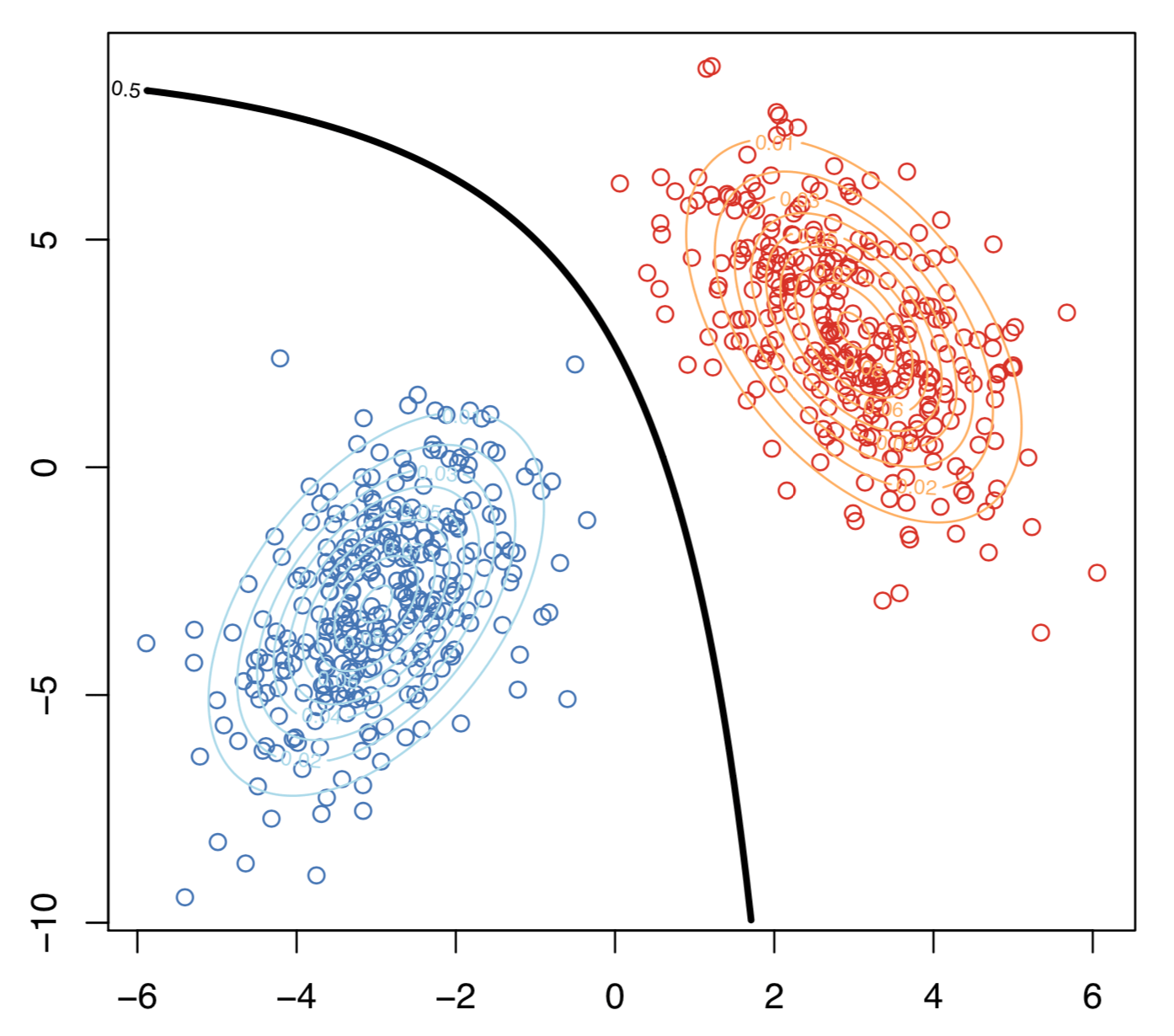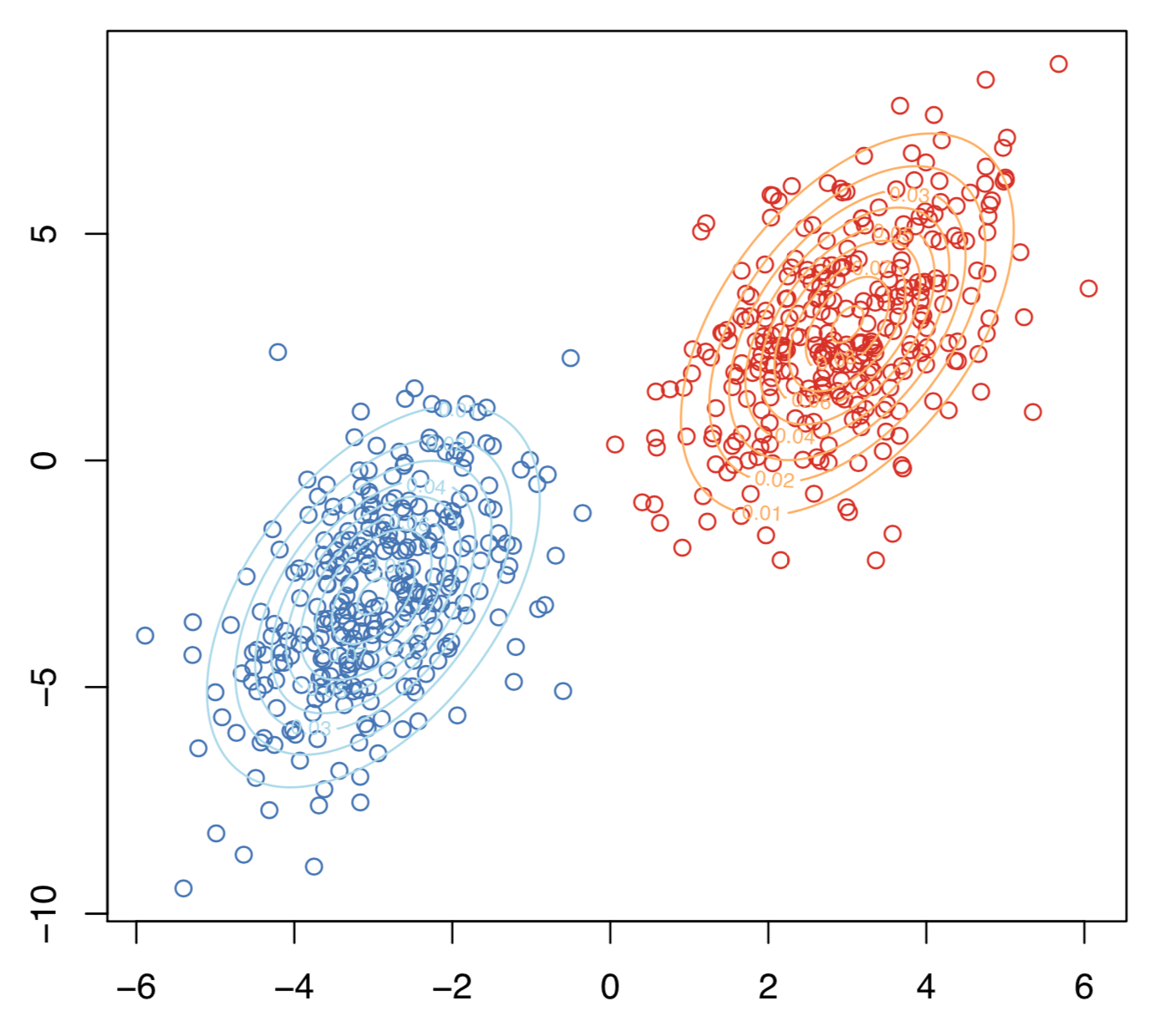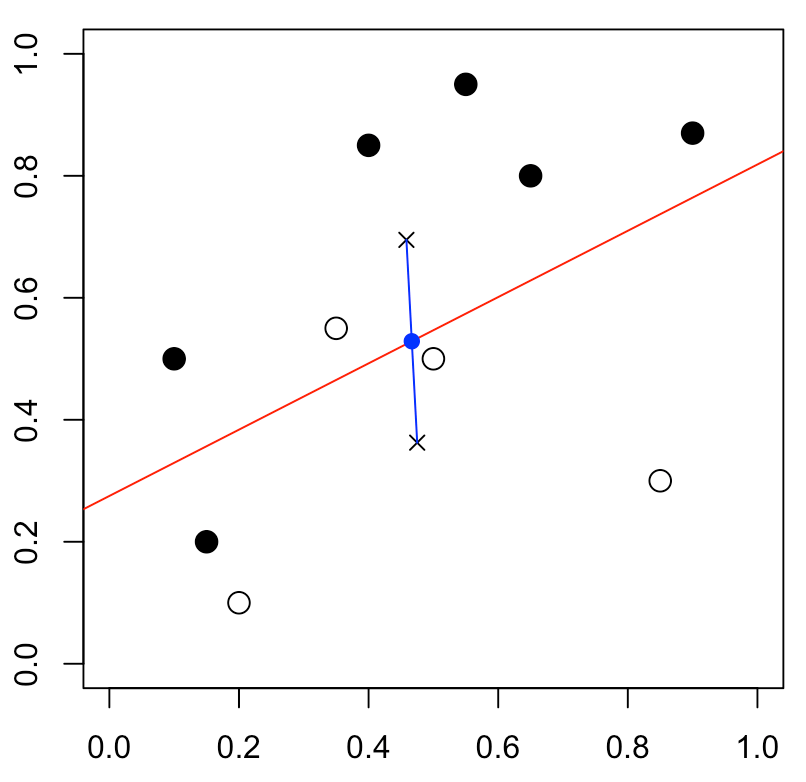# Classification from scratch, linear discrimination 8/8

June 6, 2018
By

(This article was first published on R-english – Freakonometrics, and kindly contributed to R-bloggers)

Eighth post of our series on classification from scratch. The latest one was on the SVM, and today, I want to get back on very old stuff, with here also a linear separation of the space, using Fisher’s linear discriminent analysis.

## Bayes (naive) classifier

Consider the follwing naive classification rule$$m^\star(\mathbf{x})=\text{argmin}_y\{\mathbb{P}[Y=y\vert\mathbf{X}=\mathbf{x}]\}$$or$$m^\star(\mathbf{x})=\text{argmin}_y\left\{\frac{\mathbb{P}[\mathbf{X}=\mathbf{x}\vert Y=y]}{\mathbb{P}[\mathbf{X}=\mathbf{x}]}\right\}$$(where $\mathbb{P}[\mathbf{X}=\mathbf{x}]$ is the density in the continuous case).

In the case where $y$ takes two values, that will be standard $\{0,1\}$ here, one can rewrite the later as$$m^\star(\mathbf{x})=\begin{cases}1\text{ if }\mathbb{E}(Y\vert \mathbf{X}=\mathbf{x})>\displaystyle{\frac{1}{2}}\\0\text{ otherwise}\end{cases}$$and the set$$\mathcal{D}_S =\left\{\mathbf{x},\mathbb{E}(Y\vert \mathbf{X}=\mathbf{x})=\frac{1}{2}\right\}$$is called the decision boundary.

Assume that$$\mathbf{X}\vert Y=0\sim\mathcal{N}(\mathbf{\mu}_0,\mathbf{\Sigma})$$and$$\mathbf{X}\vert Y=1\sim\mathcal{N}(\mathbf{\mu}_1,\mathbf{\Sigma})$$then explicit expressions can be derived.$$m^\star(\mathbf{x})=\begin{cases}1\text{ if }r_1^2< r_0^2+2\displaystyle{\log\frac{\mathbb{P}(Y=1)}{\mathbb{P}(Y=0)}+\log\frac{\vert\mathbf{\Sigma}_0\vert}{\vert\mathbf{\Sigma}_1\vert}}\\0\text{ otherwise}\end{cases}[/latex]where $r_y^2$ is the Manalahobis distance, [latex display="true"]r_y^2 = [\mathbf{X}-\mathbf{\mu}_y]^{\text{{T}}}\mathbf{\Sigma}_y^{-1}[\mathbf{X}-\mathbf{\mu}_y][/latex]

Let [latex]\delta_y$$

be defined as$$\delta_y(\mathbf{x})=-\frac{1}{2}\log\vert\mathbf{\Sigma}_y\vert-\frac{1}{2}[{\color{blue}{\mathbf{x}}}-\mathbf{\mu}_y]^{\text{{T}}}\mathbf{\Sigma}_y^{-1}[{\color{blue}{\mathbf{x}}}-\mathbf{\mu}_y]+\log\mathbb{P}(Y=y)$$the decision boundary of this classifier is $$\{\mathbf{x}\text{ such that }\delta_0(\mathbf{x})=\delta_1(\mathbf{x})\}$$which is quadratic in ${\color{blue}{\mathbf{x}}}$. This is the quadratic discriminant analysis. This can be visualized bellow.The decision boundary is hereBut that can’t be the linear discriminant analysis, right? I mean, the frontier is not linear… Actually, in Fisher’s seminal paper, it was assumed that $\mathbf{\Sigma}_0=\mathbf{\Sigma}_1$.

In that case, actually, $$\delta_y(\mathbf{x})={\color{blue}{\mathbf{x}}}^{\text{T}}\mathbf{\Sigma}^{-1}\mathbf{\mu}_y-\frac{1}{2}\mathbf{\mu}_y^{\text{T}}\mathbf{\Sigma}^{-1}\mathbf{\mu}_y+\log\mathbb{P}(Y=y)$$ and the decision frontier is now linear in ${\color{blue}{\mathbf{x}}}$. This is the linear discriminant analysis. This can be visualized bellowHere the two samples have the same variance matrix and the frontier is## Link with the logistic regression

Assume as previously that$$\mathbf{X}\vert Y=0\sim\mathcal{N}(\mathbf{\mu}_0,\mathbf{\Sigma})$$and$$\mathbf{X}\vert Y=1\sim\mathcal{N}(\mathbf{\mu}_1,\mathbf{\Sigma})$$then$$\log\frac{\mathbb{P}(Y=1\vert \mathbf{X}=\mathbf{x})}{\mathbb{P}(Y=0\vert \mathbf{X}=\mathbf{x})}$$is equal to $$\mathbf{x}^{\text{{T}}}\mathbf{\Sigma}^{-1}[\mathbf{\mu}_y]-\frac{1}{2}[\mathbf{\mu}_1-\mathbf{\mu}_0]^{\text{{T}}}\mathbf{\Sigma}^{-1}[\mathbf{\mu}_1-\mathbf{\mu}_0]+\log\frac{\mathbb{P}(Y=1)}{\mathbb{P}(Y=0)}$$which is linear in $\mathbf{x}$$$\log\frac{\mathbb{P}(Y=1\vert \mathbf{X}=\mathbf{x})}{\mathbb{P}(Y=0\vert \mathbf{X}=\mathbf{x})}=\mathbf{x}^{\text{{T}}}\mathbf{\beta}$$Hence, when each groups have Gaussian distributions with identical variance matrix, then LDA and the logistic regression lead to the same classification rule.

Observe furthermore that the slope is proportional to $\mathbf{\Sigma}^{-1}[\mathbf{\mu}_1-\mathbf{\mu}_0]$, as stated in Fisher’s article. But to obtain such a relationship, he observe that the ratio of between and within variances (in the two groups) was$$\frac{\text{variance between}}{\text{variance within}}=\frac{[\mathbf{\omega}\mathbf{\mu}_1-\mathbf{\omega}\mathbf{\mu}_0]^2}{\mathbf{\omega}^{\text{T}}\mathbf{\Sigma}_1\mathbf{\omega}+\mathbf{\omega}^{\text{T}}\mathbf{\Sigma}_0\mathbf{\omega}}$$which is maximal when $\mathbf{\omega}$ is proportional to $\mathbf{\Sigma}^{-1}[\mathbf{\mu}_1-\mathbf{\mu}_0]$, when $\mathbf{\Sigma}_0=\mathbf{\Sigma}_1$.

## Homebrew linear discriminant analysis

To compute vector $\mathbf{\omega}$

 1 2 3 4 5 6 7 8 9 10 11 12 13  m0 = apply(myocarde[myocarde$PRONO=="0",1:7],2,mean) m1 = apply(myocarde[myocarde$PRONO=="1",1:7],2,mean) Sigma = var(myocarde[,1:7]) omega = solve(Sigma)%*%(m1-m0) omega [,1] FRCAR -0.012909708542 INCAR 1.088582058796 INSYS -0.019390084344 PRDIA -0.025817110020 PAPUL 0.020441287970 PVENT -0.038298291091 REPUL -0.001371677757

For the constant – in the equation $\omega^T\mathbf{x}+b=0$ – if we have equiprobable probabilities, use

 1  b = (t(m1)%*%solve(Sigma)%*%m1-t(m0)%*%solve(Sigma)%*%m0)/2

## Application (on the small dataset)

In order to visualize what’s going on, consider the small dataset, with only two covariates,

 1 2 3 4 5 6 7 8 9 10 11 12  x = c(.4,.55,.65,.9,.1,.35,.5,.15,.2,.85) y = c(.85,.95,.8,.87,.5,.55,.5,.2,.1,.3) z = c(1,1,1,1,1,0,0,1,0,0) df = data.frame(x1=x,x2=y,y=as.factor(z)) m0 = apply(df[df$y=="0",1:2],2,mean) m1 = apply(df[df$y=="1",1:2],2,mean) Sigma = var(df[,1:2]) omega = solve(Sigma)%*%(m1-m0) omega [,1] x1 -2.640613174 x2 4.858705676Using R regular function, we get

 1 2 3 4 5 6 7 8  library(MASS) fit_lda = lda(y ~x1+x2 , data=df) fit_lda   Coefficients of linear discriminants: LD1 x1 -2.588389554 x2 4.762614663

which is the same coefficient as the one we got with our own code. For the constant, use

 1  b = (t(m1)%*%solve(Sigma)%*%m1-t(m0)%*%solve(Sigma)%*%m0)/2

If we plot it, we get the red straight line

 1 2  plot(df$x1,df$x2,pch=c(1,19)[1+(df$y=="1")]) abline(a=b/omega,b=-omega/omega,col="red")As we can see (with the blue points), our red line intersects the middle of the segment of the two barycenters  1 2 3 4  points(m0["x1"],m0["x2"],pch=4) points(m1["x1"],m1["x2"],pch=4) segments(m0["x1"],m0["x2"],m1["x1"],m1["x2"],col="blue") points(.5*m0["x1"]+.5*m1["x1"],.5*m0["x2"]+.5*m1["x2"],col="blue",pch=19) Of course, we can also use R function  1 2 3  predlda = function(x,y) predict(fit_lda, data.frame(x1=x,x2=y))$class==1 vv=outer(vu,vu,predlda) contour(vu,vu,vv,add=TRUE,lwd=2,levels = .5)One can also consider the quadratic discriminent analysis since it might be difficult to argue that $\mathbf{\Sigma}_0=\mathbf{\Sigma}_1$

 1  fit_qda = qda(y ~x1+x2 , data=df)

The separation curve is here

 1 2 3 4 5  plot(df$x1,df$x2,pch=19, col=c("blue","red")[1+(df$y=="1")]) predqda=function(x,y) predict(fit_qda, data.frame(x1=x,x2=y))$class==1 vv=outer(vu,vu,predlda) contour(vu,vu,vv,add=TRUE,lwd=2,levels = .5)R-bloggers.com offers daily e-mail updates about R news and tutorials on topics such as: Data science, Big Data, R jobs, visualization (ggplot2, Boxplots, maps, animation), programming (RStudio, Sweave, LaTeX, SQL, Eclipse, git, hadoop, Web Scraping) statistics (regression, PCA, time series, trading) and more...

If you got this far, why not subscribe for updates from the site? Choose your flavor: e-mail, twitter, RSS, or facebook...